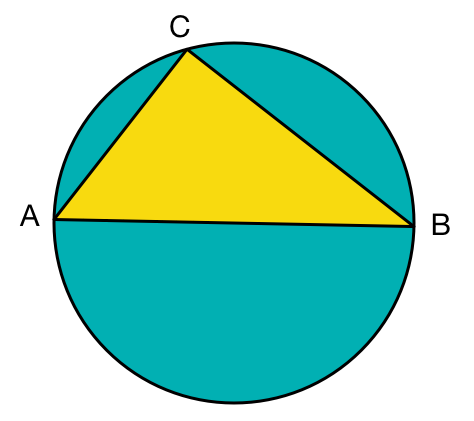# Perimeter / Radius = $2 \pi$

Geometry Level 3$AB$ is a diameter of the circle above, and $C$ is a point on the circumference such that
$\frac { \text{Area of Circle} } { \text{ Area of } \triangle ABC } = 2 \pi.$ If $\angle B < \angle A$, what is the measure of $\angle B$ in degrees?

×